# matplotlib.pyplot.xlim¶

matplotlib.pyplot.xlim(*args, **kwargs)[source]

Get or set the x limits of the current axes.

Call signatures:

left, right = xlim()  # return the current xlim
xlim((left, right))   # set the xlim to left, right
xlim(left, right)     # set the xlim to left, right


If you do not specify args, you can pass left or right as kwargs, i.e.:

xlim(right=3)  # adjust the right leaving left unchanged
xlim(left=1)  # adjust the left leaving right unchanged


Setting limits turns autoscaling off for the x-axis.

Returns: left, right A tuple of the new x-axis limits.

Notes

Calling this function with no arguments (e.g. xlim()) is the pyplot equivalent of calling get_xlim on the current axes. Calling this function with arguments is the pyplot equivalent of calling set_xlim on the current axes. All arguments are passed though.

## Examples using matplotlib.pyplot.xlim¶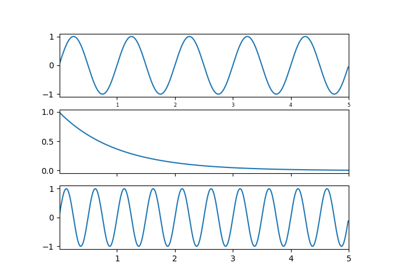Shared Axis Demo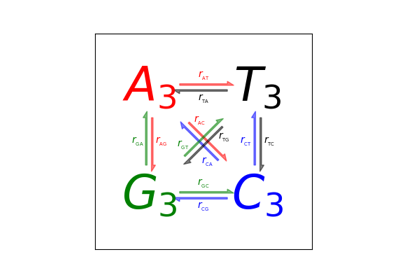Arrow Demo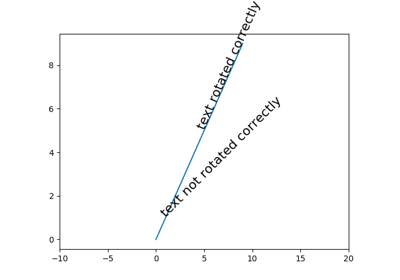Text Rotation Relative To Line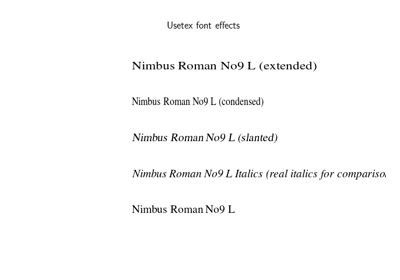Usetex Fonteffects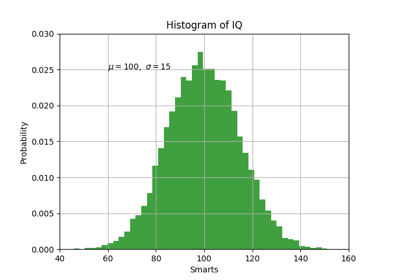Pyplot Text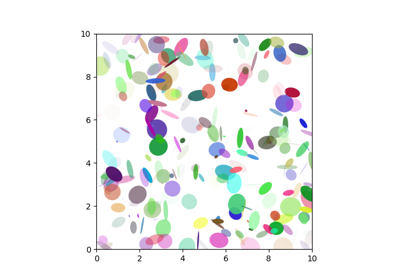Ellipse DemoFrame grabbingInteractive functions